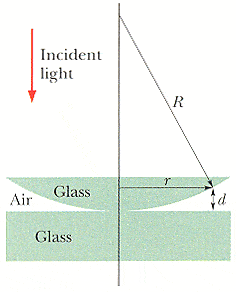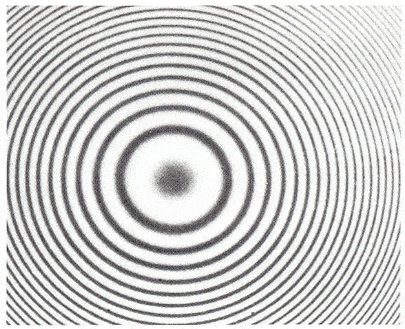# Circular Interference, Newton's Rings

doogles8654

## Homework Statement

The figure below shows a lens with radius of curvature R lying on a flat glass plate and illuminated from above by light with wavelength λ. This photo, taken from above the lens, shows that circular interference fringes (called "Newton's rings") appear, associated with the variable thickness d of the air film between the lens and the plate. The radius of curvature R of the lens is 5.0 m and the lens diameter is 19 mm.(a) How many bright rings are produced? Assume that λ = 568 nm.
rings

(b) How many bright rings would be produced if the arrangement were immersed in water (n = 1.33)?
rings

## Homework Equations

maxima in thin film interference: 2L=(m=.5)λ/n2
A of a circle = $$\pi$$r2 ?

## The Attempt at a Solution

it would seem as simple as solving the equation 2L=mλ/n2 for m when the length of the air between the curved glass and the flat glass is greatest, wouldn't that tell you how many rings you've gone through to get to the edge? But I cannot seem to figure out how large the space of air IS right at the edge....

I think one also needs to find out whether light reflected will tend to be put in phase or out of phase.

## Answers and Replies

Mentor
Light moving from a medium with a lower index of refraction to one of a higher index of refraction is phase inverted upon reflection. That is, it receives the equivalent of a π radian shift (180°), or λ/2 path length "adjustment". Add λ/2 to the gap distance.

doogles8654
but how does adding λ/2 to the gap distance help? isn't it changing all the way from the center of the whole unit to the outside?

Mentor
but how does adding λ/2 to the gap distance help? isn't it changing all the way from the center of the whole unit to the outside?

Yes, of course. You have to figure out the total gap change, and then how many times in between zero and that maximum gap amount the distance will be a suitable length to cause constructive interference. It doesn't matter what the "shape" of the change in gap is with respect to radius, as long as it's monotonically increasing over the domain.

For a given gap distance d, what is the condition for constructive interference to be observed?

doogles8654
But don't you need to know the index of refraction of the glass to figure that out?

Mentor
But don't you need to know the index of refraction of the glass to figure that out?

Nope. Everything that happens to the interfering rays in the glass happens equally to both. The only difference between the rays is that one traverses the gap and is reflected from the lower gap boundary. It is enough to know that the index of refraction of glass is greater than that of air to determine what the phase shift will be for the reflections.

EDIT: Of course, I should mention that it is necessary to know the wavelength of the light while it's in the gap.

Last edited:
doogles8654
The biggest thing to consider is whether there is a phase shift due to reflection off the bottom piece of glass right? Because that's going to decide whether the equations for the maxima and minima are 2L/$$\lambda$$=m or 2L/ $$\lambda$$ =m+.5?

Mentor
The biggest thing to consider is whether there is a phase shift due to reflection off the bottom piece of glass right? Because that's going to decide whether the equations for the maxima and minima are 2L/$$\lambda$$=m or 2L/ $$\lambda$$ =m+.5?

Right. Do you know the approximate indexes of refraction for all the materials involved (air, glass, water)?

doogles8654
So I think if i get this right, the equation for the maxima is 2L/$$\lambda$$=m? Its like the reverse of a bubble in air? It's only going to have a phase shift due to reflection once not twice. The air gap acts as the thin film. It just has a lower index of refraction than everything around it, whereas a bubble has a higher index of refraction than everything around it?

doogles8654
Right. Do you know the approximate indexes of refraction for all the materials involved (air, glass, water)?

Yeah, I can find them, but do you actually need them to find how many bright firnges there are?

Mentor
Yeah, I can find them, but do you actually need them to find how many bright firnges there are?

You only need to know their relative sizes so that you know whether or not a reflection causes a phase inversion.

doogles8654
I figured it all out!

Thanks so much for your help!! :)